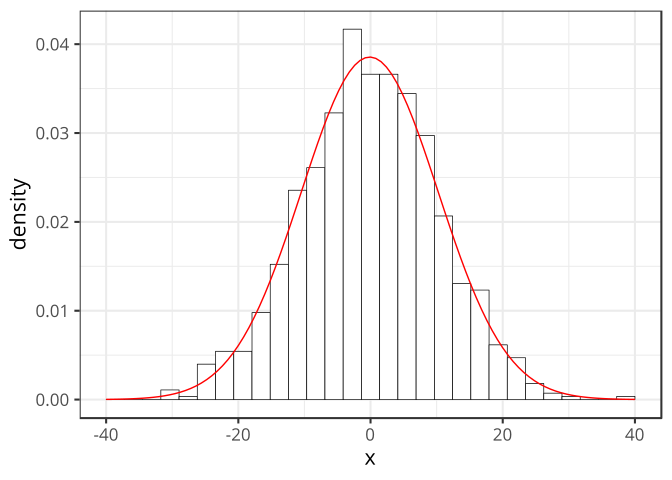# Generalized linear models (GLM)

## Introduction to GLM

Until now, we have worked with statistical analysis (anova, lm) that supposed a normal distribution of the response variable.## Normal distribution

Data: Daily maximum temperature in Roskilde between 2015-01-01 and 2015-12-18.

``````temperature <- read_csv("data/temp_roskilde_2015.csv")
temperature <- janitor::clean_names(temperature)
temperature\$cet <- as.Date(temperature\$cet, format = "%Y-%m-%d")``````

## The data

cet max_temperature_c mean_temperature_c min_temperature_c dew_point_c mean_dew_point_c min_dewpoint_c
1 16436.00 6.00 4.00 3.00 5.00 4.00 2.00
2 16437.00 9.00 7.00 4.00 7.00 3.00 0.00
3 16438.00 6.00 3.00 2.00 3.00 2.00 0.00
4 16439.00 4.00 2.00 1.00 2.00 -1.00 -3.00
5 16440.00 4.00 2.00 0.00 3.00 2.00 0.00
6 16441.00 3.00 1.00 -1.00 3.00 1.00 0.00
7 16442.00 6.00 3.00 1.00 4.00 2.00 0.00
8 16443.00 7.00 4.00 3.00 6.00 4.00 2.00
9 16444.00 7.00 5.00 3.00 5.00 2.00 1.00
10 16445.00 10.00 7.00 3.00 9.00 3.00 -2.00

``````ggplot(temperature, aes(x = cet, y = mean_temperature_c)) +
geom_point() + geom_smooth(method = "loess") +
scale_x_date()``````